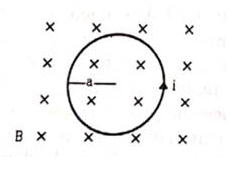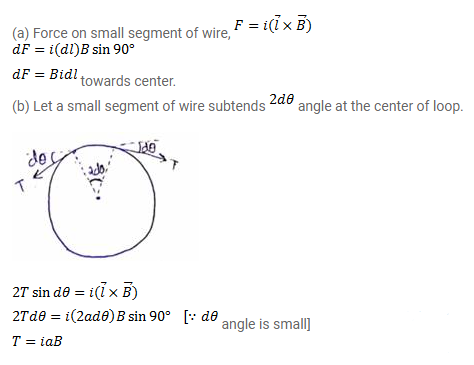# Figure shows a circular wire-loop of radius a,

Question:

Figure shows a circular wire-loop of radius a, carrying a current i, placed in a perpendicular magnetic field B.

(a) Consider a small part dl of the wire. Find the force on this part of the wire exerted by the magnetic field.

(b) Find the force of compression in the wire.Solution: